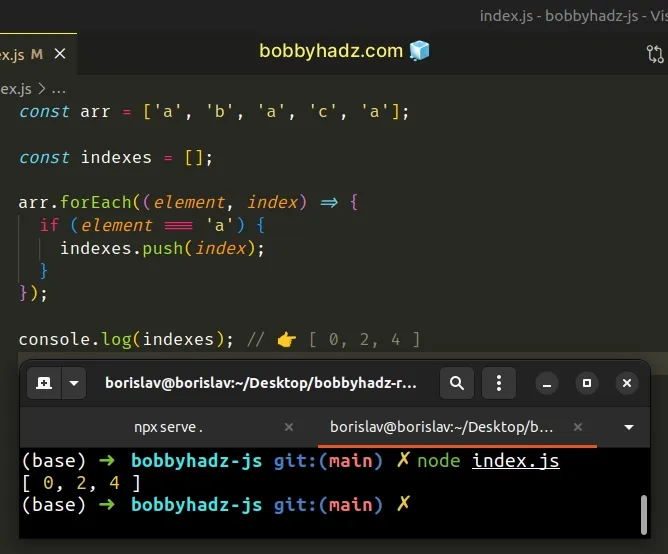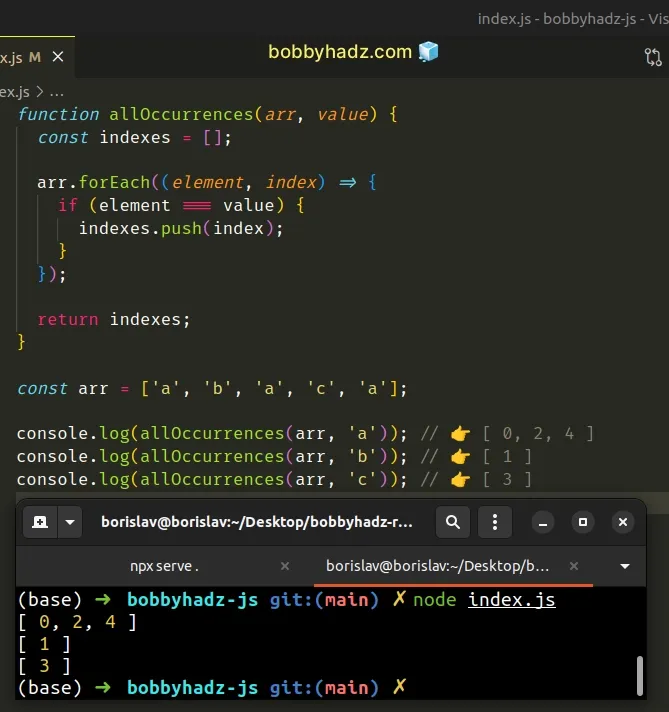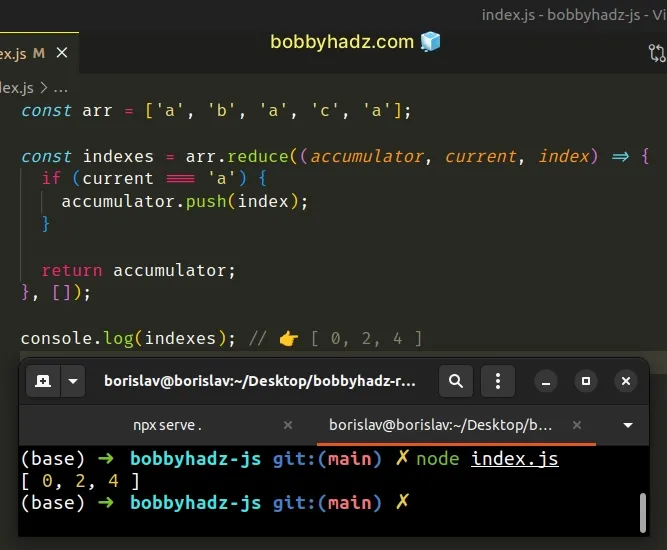# Find the indexes of all Occurrences of Element in JS ArrayLast updated: Dec 21, 2022
3 min## #Find the indexes of all Occurrences of Element in JS Array

To get the index of all occurrences of an element in an array:

1. Declare a new variable and initialize it to an empty array.
2. Use the `forEach()` method to iterate over the original array.
3. Check if each element is equal to the specified value.
4. Push the matching elements into the new array.
index.js
```Copied!```const arr = ['a', 'b', 'a', 'c', 'a'];

const indexes = [];

arr.forEach((element, index) => {
if (element === 'a') {
indexes.push(index);
}
});

console.log(indexes); // 👉️ [ 0, 2, 4 ]
``````We declared a new variable and initialized it to an empty array.

The `indexes` variable will store the indexes of all occurrences of the element in the array.

The function we passed to the Array.forEach method gets called with each element in the array.

On each iteration, we check if the current element is equal to the specified value.

If the condition is met, we push the current index into the `indexes` array.

JavaScript indexes are zero-based in JavaScript, so the first element in the array has an index of `0` and the last element has an index of `arr.length - 1`.

If you have to do this often, define a reusable function.

index.js
```Copied!```function allOccurrences(arr, value) {
const indexes = [];

arr.forEach((element, index) => {
if (element === value) {
indexes.push(index);
}
});

return indexes;
}

const arr = ['a', 'b', 'a', 'c', 'a'];

console.log(allOccurrences(arr, 'a')); // 👉️ [ 0, 2, 4 ]
console.log(allOccurrences(arr, 'b')); // 👉️ [ 1 ]
console.log(allOccurrences(arr, 'c')); // 👉️ [ 3 ]
``````The function takes an array and a value as parameters and returns all of the indexes of the value in the array.

Alternatively, you can use the `Array.reduce()` method.

## #Find the Index of all Occurrences of an Element in Array using `reduce()`

This is a three-step process:

1. Use the `Array.reduce()` method to iterate over the array.
2. Check if the current element is equal to the specified value.
3. Push the indexes of the matching elements into the `accumulator` array.
index.js
```Copied!```const arr = ['a', 'b', 'a', 'c', 'a'];

const indexes = arr.reduce((accumulator, current, index) => {
if (current === 'a') {
accumulator.push(index);
}

return accumulator;
}, []);

console.log(indexes); // 👉️ [ 0, 2, 4 ]
``````The function we passed to the Array.reduce method gets called for each element in the array.

We initialized the `accumulator` variable to an array because that's what we passed as the second argument to the `reduce()` method.

The value we return from the callback function gets passed as the `accumulator` on the next iteration.

On each iteration, we check if the current element is equal to a specific value.

If the condition is met, we push the current index into the `accumulator` array.

Otherwise, we return the `accumulator` array as is.

After the last iteration, the `indexes` array stores the index of all occurrences of the element in the array.

## #Find the Index of all Occurrences of an Element in Array using `map()`

You can also use the `map()` and `filter()` methods to find the index of all occurrences of an element in an array.

index.js
```Copied!```const arr = ['a', 'b', 'a', 'c', 'a'];

const value = 'a';

const indexes = arr
.map((element, index) => (element === value ? index : -1))
.filter(element => element !== -1);

console.log(indexes); // 👉️ [ 0, 2, 4 ]
``````

The function we passed to the Array.map() method gets called with each element in the array.

The `map()` method returns a new array containing the values returned from the callback function.

index.js
```Copied!```const arr = ['a', 'b', 'a', 'c', 'a'];

const value = 'a';

const indexes = arr.map((element, index) =>
element === value ? index : -1,
);

console.log(indexes); // 👉️ [ 0, -1, 2, -1, 4 ]
``````

For each element that doesn't meet the condition, we return `-1`.

The last step is to use the `Array.filter()` method to remove the `-1` values from the array.

index.js
```Copied!```const arr = ['a', 'b', 'a', 'c', 'a'];

const value = 'a';

const indexes = arr
.map((element, index) => (element === value ? index : -1))
.filter(element => element !== -1);

console.log(indexes); // 👉️ [ 0, 2, 4 ]
``````

The function we passed to the Array.filter method gets called with each element in the array.

The `filter()` method returns a new array that only contains the elements that meet the condition.